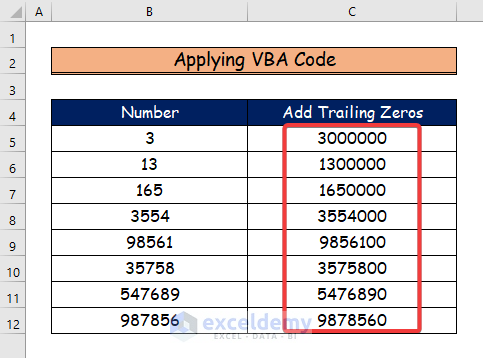# How to Add Trailing Zeros in Excel (2 Easy Ways)

Get FREE Advanced Excel Exercises with Solutions!

When adding trailing zeroes to the right of cell contents, you sometimes need to normalize the numbers so that they are all the same length. Other times, however, you merely need to add trailing zeroes without taking into account the constant length of all specified cells. In this article, we will show you how to add trailing zeros in Excel.

## How to Add Trailing Zeros in Excel: 2 Handy Approaches

You will discover two extremely practical ways to add trailing zeros in Excel using VBA code and the manual REPT and LEN functions in the two approaches that follow. Let’s suppose we have a sample data set.### 1. Using the REPT and LEN Functions to Add Trailing Zeros in Excel

In this first method, you will learn how to add trailing zeros in Excel by using the REPT and LEN functions. Follow the outlined steps below to do the task.

Step 1:

• Firstly, select cell C5.
• And, write down the following formula to add trailing zeros.

`=B5&REPT("0",7-LEN(B5))`Step 2:

• Secondly, press Enter.
• Here, cell C5 represents the trailing zero for the first number.
• Now, use the Fill handle tool and drag it down from cell C5 to C12.Formula Breakdown

• =B5&REPT(“0”,7-LEN(B5)): AT first we will select the B5 cell, and the &allows us to append a value to the value in cell B5.
• This formula contains the REPT function which shows us the next parameter required that we will repeat as 0 in cell B5 to get our 7-digit numbers.
• Finally, the LEN function determines the number of digits in column B5.

Step 3:

• Therefore, we get the following results for the other cells of trailing zeros.### 2. Applying VBA Code to Add Trailing Zeros in Excel

In this last section, we will generate a VBA code utilizing the Developer tab to add trailing zeros in Excel.

Step 1:

• At first, we will use the Developer tab.
• Then, we will select the Visual Basic command.Step 2:

• Here, the Visual Basic window will open.
• After that, from the Insert option, we will choose the new Module to write a VBA code.Step 3:

• Now, paste the following VBA code into the Module
• To run the program, click the “Run” button or press F5.
``````Sub Add_Trailing_Zeros()
'Declare variables
Dim Mysheet As Worksheet
Set Mysheet = Worksheets("Add Trailing Zeros")
'Add trailing zeros from cell C5 to C12
For x = 5 To 12
Mysheet.Range("C" & x) = Mysheet.Range("B" & x) & WorksheetFunction.Rept("0", 7 - Len(Mysheet.Range("B" & x)))
Next x
End Sub``````VBA Code Breakdown

• Firstly, we call our Sub Procedure Add_Trailing_Zeros.
• Then, we declare our variable as Worksheet.
• Besides, we set our worksheet name as Add Trailing Zeros.
• Finally, we select the range from cell C5 to C12 to add  trailing zeros using For x = 5 To 12 and Mysheet.Range(“C” & x) = Mysheet.Range(“B” & x) & WorksheetFunction.Rept(“0”, 7 – Len(Mysheet.Range(“B” & x).

Step 4:

• The outcomes for the other cells with trailing zeros are as follows.

##You may download the following Excel workbook for better understanding and practice yourself.

## Conclusion

In this article, I’ve covered 2 handy methods to add trailing zeros in Excel. I sincerely hope you enjoyed and learned a lot from this article. Additionally, if you want to read more articles on Excel, you may visit our website, Exceldemy. If you have any questions, comments, or recommendations, kindly leave them in the comment section below.

## What is ExcelDemy?

ExcelDemy Learn Excel & Excel Solutions Center provides free Excel tutorials, free support , online Excel training and Excel consultancy services for Excel professionals and businesses. Feel free to contact us with your Excel problems.Bishawajit Chakraborty

I'm Bishawajit Chakraborty. Hello. I graduated from Rajshahi University of Engineering & Technology (RUET) with a degree in Mechanical Engineering. I'm working with ExcelDemy as a Content Developer for Excel & VBA. You can visit our website, Exceldemy if you'd like to read my published articles on MS Excel and VBA.

We will be happy to hear your thoughtsAdvanced Excel Exercises with Solutions PDF